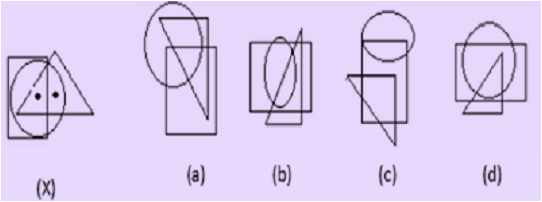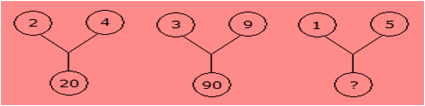# SilverZone - IOM PDF Sample Papers for Class 8

Resources:

Class 8 sample paper & practice questions for International Olympiad of Mathematics (IOM) level 1 are given below. Syllabus for level 1 is also mentioned for these exams. You can refer these sample paper & quiz for preparing for the exam.Q.1 Q.2 Q.3 Q.4 Q.5 Q.6 Q.7 Q.8 Q.9 Q.10
 Q.1 Select the figure which satisfies the same conditions of placement of the dots as in fig. (X).a) a b) b c) c d) d
 Q.2 Find the missing character from among the given alternatives:a) 75 b) 20 c) 25 d) 26
 Q.3 If in the word 'DISTURBANCE', the first letter is interchanged with the last letter, the second letter is interchanged with the tenth letter and so on, which letter would come after the letter T in the newly formed word? a) N b) C c) S d) E
 Q.4 A geometric representation showing the relationship between a whole and its parts is a: a) Pie Chart b) Histogram c) Bar Graph d) Pictograph
 Q.5 The solution of which of the following equations is a fraction not an integer? a) 3x + 2 = 5x + 2 b) 4x - 18 = 2 c) 4x - 7 = x + 2 d) 5x - 9 = x + 4
 Q.6 A four-digit number aabb is divisible by 55. Then possible value(s) of b is/are: a) 0 and 2 b) 2 and 5 c) 0 and 5 d) Can't be determined
 Q.7 Which is the smallest square number that is divisible by each of the number 4, 9 and10? a) 900 b) 810 c) 800 d) 920
 Q.8 A car needs 54 litre of diesel for covering a distance of 297 km. The diesel required by the car to cover a distance of 550 km is: a) 100 litres b) 50 litres c) 25.16 litres d) 25 litres
 Q.9 Sum of all interior angles of a parallelogram is ___________. a) 180o b) 360o c) 540o d) 240o
 Q.10 Find √0.9 × √1.6 =? a) 0.12 b) 3.4 c) 0.75 d) 1.2Sample PDF of SilverZone - International Olympiad of Mathematics (IOM) PDF Sample Papers for Class 8: